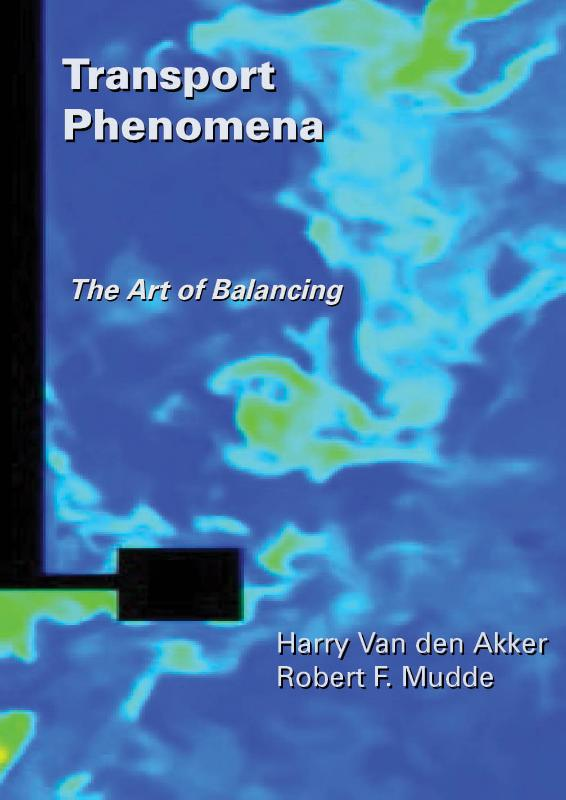# Transport phenomena

Taal: Engels

## € 51,40

Transport Phenomena The Art of Balancing First Edition 2014 In this textbook, the transport and transfer processes of heat, mass and momentum are treated in terms of their analogy. The processes are described with the help of macro and micro balances which in many cases lead to differential equations. This way, the textbook also prepares for Computational Fluid Dynamics techniques. The topics of the various chapters of the textbook are: Balances: shape and recipe, mass balance, residence time distribution, energy and heat balances, Bernoulli equation, momentum balances Mechanisms, non-dimensional numbers, forces: molecular transport, dimensional analysis, forces on immersed objects Heat transport: steady-state and unsteady conduction, Newton's Cooling Law, the general heat transport equation, convective heat transport (forced and free convection), heat exchangers, radiant heat transport Mass transport: mutual and unilateral, steady-state and unsteady diffusion, the general mass transport equation, partition coefficient for mass transfer across a phase interface, convective mass transport, wet bulb temperature Fluid mechanics: flow meters, pressure drop over straight pipes and pipeline systems, packed bed, laminar flow of Newtonian and non-Newtonian fluids, the Navier-Stokes equations The leading idea behind this textbook is to train students in solving problems where transport phenomena are key. To this end, the textbook comprises almost 80 problems with solutions.
ISBN
9789065623584
Vorm
Gebonden
Uitgever